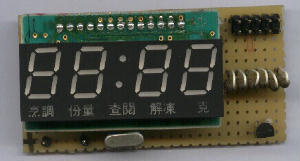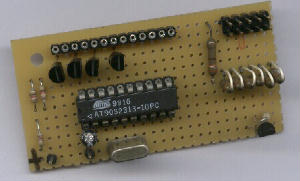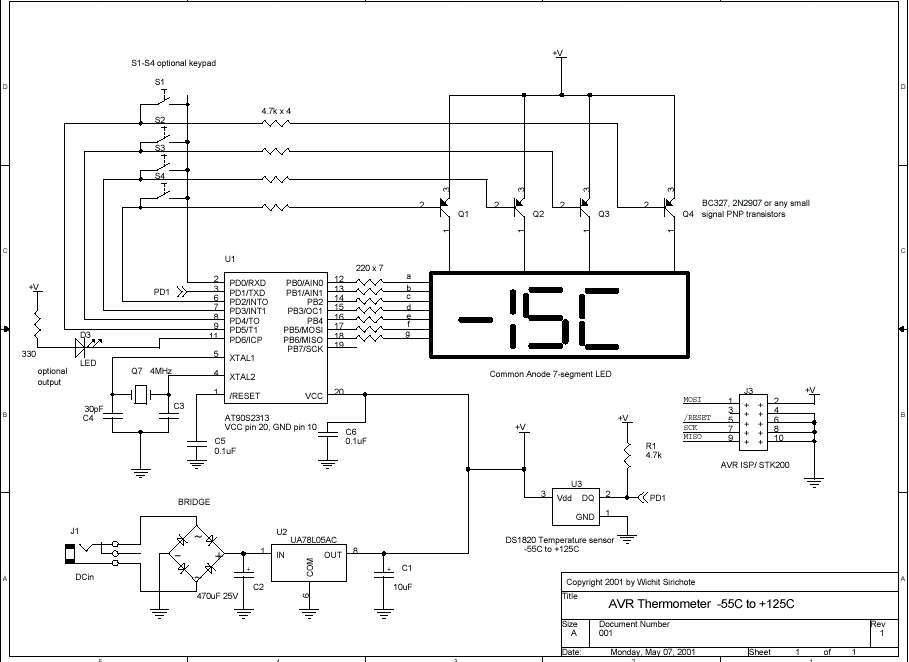AVR Thermometer

Wichit Sirichote, kswichit@kmitl.ac.th

Build a simple digital thermometer using AT90S2313 and DS1820. Download the hex code directly to the chip with ISP cable. The thermometer is capable of measuring temperature from -55C to +125C with 1 degreeC resolution.

Introduction

I bought the LED module from BanMor' last week, just 30Baht. The module provide a prewired multiplex of 4-digit common anode LED, that's great. See the soldering pad of these signal in the 1st picture below. I thought, my friend gave me the AT90S2313 chip, and with a simple homemade ISP cable and a free software AVR ISP downloader, so we don't need the device programmer. Also I got the DS1820 from my friend. So I spent my freetime weekend putting them together, "the AVR Thermometer".  The 2nd picture shows a sample circuit using universal PCB, see the upper right,  it was 10-pin header for STK200 compatible downloader. Of course the LED module was designed for CLOCK display, so you may interested in writing C program for both Clock and Temperature display after built this project.Circuit

The circuit diagram is straight forward, similar to 89C2051 Clock Circuit. PORTB sinks forward current of each LED segment through a 220 Ohms resistor. The common anode pin for each digit is controlled by PORTD, i.e., PD2-PD5. The PNP transistor can be any types of small signal transistor. S1-S4 are optional keypad. Also PD6 is optional output. All of LEDs are driven with sink current.

The temperature sensor chip, DS1820 is connected to PD1 with a 4.7k pull-up resistor. The example of circuit uses only one sensor, you may tie multiple sensors on the same line and modify the program to read it with the help of internal chip ID.

J3 is AVR ISP/STK200 compatible downloader. See the pin designation and build the cable from Experimenting the AT90S8535 with CodeVisionAVR C Compiler

The software for download the hex code, thermo.hex to the 2313 chip, can get from ATMEL FTP website here ATMEL AVR ISP.

Software

The source porgram was written in C language and compiled with AVR CodeVision C compiler. Actually my first try used the AT90S8535, then I moved the C code from 8535 to 2313 with a bit modification.

The program was foreground and background running, or interrupt driven. The background task is forever loop scanning 4-digit LED.

main()
{
while (1)
{
scanLED();  //  run background task forever
}
}

The function scan display was borrowed from 89C2051 Clock controller. (Actually no need any modifications if we use a preprocessor to define such I/O port.)

#define segment PORTB
#define LED_digit PORTD

void scanLED() /* scan 4-digit LED and 4-key switch, if key pressed key = 0-3
else key = -1 */
// adapted from 89C2051 project  if needs scan key, find one bit input port
{
char i;
digit = 0x20;
key = -1;
for( i = 0; i < 4; i++)  /* 4-DIGIT scanning */
{
LED_digit = ~digit;  /* send complement[digit] */
segment = ~heat[i];  /* send complement[segment] */
delay_ms(1);         /* delay a while */
segment = 0xff;        /* off LED */
//   if ((PORTD & 0x10) == 0) /* if key pressed P3.4 became low */
//      key = i;       /* save key position to key variable */
digit>>=1;        /* next digit */
}
}

for-loop statement provides 4-cycle scanning from digit0 to digit3. First we activate digit control line by sending complement of digit variable. Follow with segment data from heat[i] array. The delay 1ms provides enough time for electron to jump back to ground state converting to electromagnetic radiation. Then turn off before changing digit. The digit data is then shifted to the right one bit. Similarly for the next digit.

The foreground task is entered by timer0 interrupt every 1/15 s. Timer0 get the internal clock from 4MHz/1024 or 3906Hz. So with an 8-bit timer0 counting up from 0 to 255 and set interrupt flag from 255 to 0 transition, the rate of interrupt will be 3906Hz/256 or 15Hz.

I used a global variable "tick" for counting a number of interrupt entering. See below, when tick equal to 15 or one second has elapsed then function read_temp() will be executed.

// Timer 0 overflow interrupt service routine
// timer interrupt every 1/15 sec provides foreground task to be run periodically.

interrupt [TIM0_OVF] void timer0_ovf_isr(void)
{
switch (++tick){
case 15: tick = 0;
}
}

When read_temp() function has entered, I used another global variable for couting up task that needs time
more than a second. The xtimer variable will count up until reachs 5, or 5s, it will read data from DS1820, performs 5-point moving average and convert the value to 7-segment pattern then.

{
if(++xtimer1 >=5)
{
xtimer1 = 0;
segment = 0xff; // turn segment off
T =  ds1820_temperature_10(0)/10;     // read DS1820 every 5 sec.
LPF();    // enter digital filter providing slowly change of temperature.
heatToBuffer();         // convert it
}
}

The function ds1820_temperature_10(0) was provided by CodeVision library.

See below what function of LPF() is;

LPF()      // performs five-point moving average
{
X5=X4;
X4=X3;
X3=X2;
X2=X1;
X1= T;
T = (X1+X2+X3+X4+X5)/5;
}

T is computed from 5-point consecutive data.

The heatToBuffer() is the function that converts signed number T to 7-segment LED pattern.

void heatToBuffer()
{
if(T<0){
heat = 0x40;  // yes, negative, put -
heat = 0x39; // C

T = abs(T);   // get only amplitude
heat =  convert[T%10];
heat = convert[T/10];
if (heat == 0x3f)
heat = 0; // off msd
}
else
{
heat = 0x39; // C

heat = convert[T/100];
temp = T%100;
heat = convert[temp%10];
heat = convert[temp/10];
// off msd
if (heat == 0x3f)
{
heat = 0;
if(heat == 0x3f)
heat = 0;
}
}
}

Reading data from 1-wire interface device, DS1820, takes about 0.2s, so when the program enter 1-wire connecting, the display will turn off, say 0.2s. Also the function that reads DS1820 will disable any interrupt, so we then loss such period of time.